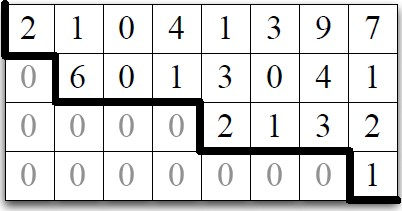# Linear Algebra - Row Space of a matrix

## Theorem

### Echelon

If a matrix is in echelon form, the nonzero rows form a basis for the row space.

Applying elementary row-addition operations does not change the row space.

## How to

### find a basis

To find basis for row space of a matrix A, iteratively transform A into a matrix B

• in echelon form
• with no zero rows
• whose row space is the same as that of A.

See Gaussian elimination - Finding a basis for the computation

Discover MoreLinear Algebra - (Gaussian|Common) Elimination

Method illustrated in Chapter Eight of a Chinese text, The Nine Chapters on the Mathematical Art, that was written roughly two thousand years ago. Rediscovered in Europe by Isaac Newton (England) and Michel...Linear Algebra - Find intersection of geometric objects

Find intersection of geometric objects Find the intersection of the plane spanned by [1, 0, 0] and [0, 1,−1] the plane spanned by [1, 2,−2] and [0, 1, 1] The orthogonal complement in of...Linear Algebra - Rows of a Matrix

Let M, A, and B be matrices, if MA = B where M is then Row A = Row B. therefore change to row causes no change in row space therefore basis for changed rowlist is also a basis for original rowlist....Linear System - Echelon Matrix

The Echelon form is a generalization of triangular matrices. An matrix A is in echelon form if it satisfies the following condition: for any row, if that row’s first nonzero entry is in position...